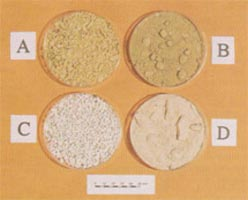Steps to test gypsum purity:

1. Determine SO3 and then divide by 0.8333 to get SO4,
2. Determine CaO and then divide by 1.4 to get Ca,
3. After checking that % SO4 x 40/96 = % Ca then:
a. Add % SO4 and % Ca
b. Divide by 4 to get theoretical LOI.
c. Multiply by 1.25 to give gypsum, Calcium Sulphate (CaSO4.2H2O) purity.

If line 3 doesn’t add up then calculate gypsum purity from Sulphate content (not calcium as some of it is likely to present as CaCO3).Other purity methods of testing:
a) Purity of gypsum is calculated by dividing Sulphur % by 0.186 (i.e. pure gypsum is 18.6% Sulphur). Sulphur is determined by the method specified in the report , that is by addition of hydrochloric acid and water then boiled for 30 minutes. Concentration of Sulphur is then determined using ICP against Standard.
b) if the product is of high quality virgin mined product with out organics in it and you have results moisture content for free and combined moisture, and

c) If the max theoretical amount of Combined water present in the sample is < 20.93, you can calculate the Purity % using the following formula, c) % Purity = (% Combined water/20.93)×100 You have visited the REGYP News Room, for more info please visit the full REGYP website www.regyp.com.au , or call 1300 473 497 for more information.